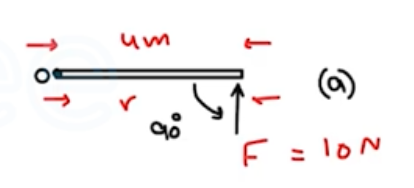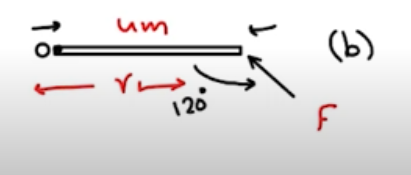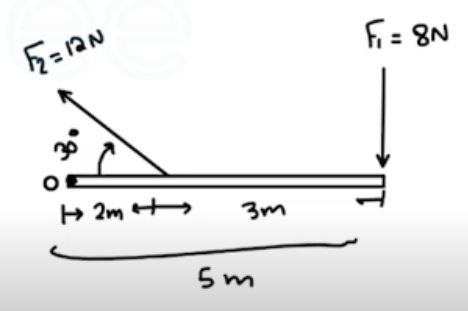Need Help?

Subscribe to Physics 1

###### \${selected_topic_name}
• Notes

$\begin{array}{l}{\text { Calculate the torque (magnitude and direction) about point }} \\ {\text { O due to the force } \overrightarrow{\boldsymbol{F}} \text { in each of the cases sketched in Fig. El0.1. In }} \\ {\text { each case, the force } \overrightarrow{\boldsymbol{F}} \text { and the rod both lie in the plane of the }} \\ {\text { page, the rod has length } 4.00 \mathrm{m}, \text { and the force has magnitude }} \\ {F=10.0 \mathrm{N} \text { . }}\end{array}$(a) $\tau=F L$

$L=r \sin \theta=4 \sin 90=4 m$

$\tau=10 * 4=40 \mathrm{N.m}$(b) $\tau=F L$

$L=r \sin \theta=4 \sin 120=3.464 \mathrm{m}$

$\tau=10 *3.464=34.6 N.m$

$\begin{array}{l}{\text { Calculate the net torque about point } O \text { for the two forces }} \\ {\text { applied as in Fig. E10.2. The rod and both forces are in the plane of }} \\ {\text { the page. }}\end{array}$$\tau=F L$

$L=r \sin \phi$

$\tau_{1}=-F_{1} L_{1}=-(8)(5)=-40 \mathrm{N} \cdot \mathrm{m}$

$\tau_{2}=+F_{2} L_{2}=(12)(2) \sin 30=12 \mathrm{N} \cdot \mathrm{m}$

$\sum\tau=\tau_{1}+\tau_{2}$

$=-40+12=-28 N \cdot m$

$\begin{array}{l}{\text { A metal bar is in the } x y \text { -plane with one end of the bar at the }} \\ {\text { origin. A force } \overrightarrow{\boldsymbol{F}}=(7.00 \mathrm{N}) \hat{\boldsymbol{i}}+(-3.00 \mathrm{N}) \hat{\boldsymbol{j}} \text { is applied to the bar }} \\ {\text { at the point } x=3.00 \mathrm{m}, y=4.00 \mathrm{m} . \text { (a) In terms of unit vectors } \hat{\boldsymbol{i}}} \\ {\text { and } \hat{\boldsymbol{j}}, \text { what is the position vector } \overrightarrow{\boldsymbol{r}} \text { for the point where the force is }} \\ {\text { applied? (b) What are the magnitude and direction of the torque }} \\ {\text { with respect to the origin produced by } \overrightarrow{\boldsymbol{F}} ?}\end{array}$

$\vec{r}=3 \hat i+4 \hat{j}$

${\tau=\vec{r} \times \vec{F}=[(3 \hat j+4 \hat j)] \times[(7 \hat{i}+(-3 \hat{j}))]}$

$\tau=-9 \hat{k}+-28- \hat k=-37 \ N.m \ \hat k$